Courses

# Magnetic Properties of Materials Civil Engineering (CE) Notes | EduRev

## Civil Engineering (CE) : Magnetic Properties of Materials Civil Engineering (CE) Notes | EduRev

The document Magnetic Properties of Materials Civil Engineering (CE) Notes | EduRev is a part of the Civil Engineering (CE) Course Engineering Physics - Notes, Videos, MCQs & PPTs.
All you need of Civil Engineering (CE) at this link: Civil Engineering (CE)

Magnetic Properties of Materials

Classification of magnetic materials
Magnetic materials can be classified in to five types on the basis of magnetic dipoles.
(1) Diamagnetic materials
(2) Paramagnetic materials
(3) Ferromagnetic materials
(4) Antiferromagnetic materials
(5) Ferrimagnetic materials

Diamagnetic Materials:
Diamagnetic materials are the substances which become weakly magnetized in the direction opposite to that of the applied field. They do not posses permanent magnetic dipoles. The magnetic dipole moment of the atoms of the material is zero. Bismuth, Gold, Water are the few examples for diamagnetic materials. When diamagnetic materials are placed in the magnetic field they expel the flux lines. A perfect diamagnet expels all the flux lines from the interior of the material.

Langevin’s theory of Diamagnetic Materials:
Langevin, in 1905, explained diamagnetism from the electron theory of matter. The atoms in diamagnetic materials contain even number of electrons. The electrons in such atoms are paired. The electrons in each pair have orbital motion as well as spin motions in opposite sense. The resultant magnetic moment of the atom is thus zero. When diamagnetic materials are placed in the magnetic field, the magnetic field does not tend to align the atoms (dipoles) of the substance in the direction of the field. But the field modifies the motions of the electrons in orbits which are equivalent to tiny current loops. The Lenzs law acts in the atomic scale. The electron moving in a direction so as to produce a magnetic field in the same direction as the external field is slowed down, while the other accelerated. Hence atom acquires an effective magnetic moment which is opposite to the applied magnetic field. It is not affected by thermal agitations and hence independent of temperature. Diamagnetism is present in all magnetic materials but it is more prevalent in case of the Diamagnetic materials. Hence it is universal property of matter. Langevin was able to derive an expression for the diamagnetic susceptibility and is given by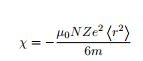Here χ is diamagnetic Susceptibility, µ0 is permeability of free space, e is electronic charge, m is the mass of the electron, ­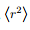is the mean square radius of the electrons orbit, N is the number of atoms per unit volume of the material and Z is the number of electrons in the atom.

Paramagnetic Materials:
Paramagnetic materials posses permanent magnetic dipoles. When such materials are placed in the magnetic field they get weakly magnetized in the direction of the applied magnetic field. Platinum, Aluminium, Manganese are the few examples of paramagnetic materials. In paramagnetic materials the interaction between the neighboring dipoles is extremely week.

Langevin’s theory of Paramagnetism:
Langevin explained paramagnetism on the basis of, atoms or molecules of a paramagnetic material. Paramagnetic materials have a net intrinsic permanent magnetic moment due to the spin and orbital motions of electrons in it. In the absence of the external magnetic field all dipoles tend to orient in random directions due to thermal agitation and hence the net magnetic moment of the material is zero.
When paramagnetic materials are placed in the magnetic field, the dipoles experience torque and they tend to align in the direction of applied field. The average alignment produced give rise to the net magnetic moment of the material in the direction of the applied field.
The paramagnetism is a function of temperature. Higher the temperature more will the randomness in the orientations of the dipoles due to thermal agitations. Hence the net dipole moment decreases with temperature. The expression for paramagnetic susceptibility as derived by Langevin is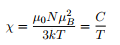χ is the paramagnetic susceptibility, µ0 is the permeability of free space, k is Boltzmann constant, T is absolute temperature, µB is the Bohr magneton and N is the number of molecules per unit volume of the material. Here C is a constant called Curie constant given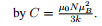Ferromagnetic Materials:
Ferromagnetic materials can be strongly magnetized by relatively weaker magnetic fields. Materials composed of elements Iron, Nickel and Cobalt exhibit ferromagnetism. In ferromagnetic materials the interaction between the neighboring dipoles is very strong. This interaction is called spin exchange interaction.

Weiss molecular field theory of Ferromagnetism:
In the year 1907 Weiss proposed a theory for susceptibility of ferromagnetic materials. The Weiss theory is based on the following assumptions.
(1) The neighboring dipoles in ferromagnetic materials interact and they group together to form ferromagnetic domains. Each domain is magnetized to saturation. In the absence of external magnetic field, since the direction of magnetization of different domains is different, the average resultant magnetization of ferromagnetic material is zero.
(2) The cause of spontaneous magnetization within each domain is the existence of the molecular field which tries to produce a parallel alignment of the dipoles.

Based on the above assumptions Weiss was able to derive an expression for ferromagnetic susceptibility as a function of excess of temperature which is given by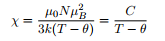Here χ is Ferromagnetic Susceptibility, C is Curie constant, T is the temperature of the substance in absolute scale called the Curie temperature.

For temperatures less than ferromagnetic Curie temperature the dipole-dipole interactions are very strong which overcome the thermal agitations. Hence the susceptibility is very high. But for temperature higher than ferromagnetic Curie temperature the violent thermal agitations overcome dipole interactions and the susceptibility varies inversely as excess of temperature and the material is transformed into paramagnetic phase.

During the magnetization of the ferromagnetic specimen by applying external magnetic field the magnetization of the specimen takes place either due to the rotation of domains magnetic moments under applied strong magnetic fields

Hysteresis in Ferromagnetic Materials (B-H Curve)
During the magnetization and demagnetization of the specimen the induction flux density (B) lags behind the applied magnetic field strength (H) and is called ferromagnetic hysteresis. B-H curve is a plot of the induction flux density versus the applied field strength during magnetization and demagnetization of a ferromagnetic specimen.
The origin O represents the initially unmagnetised specimen and zero magnetic intensity. As H is increased B also increases and saturation (Bs) is reached (curve oa). At saturation all domains are oriented in the same direction as the applied field. If H is reduced B decreases. But when H reduces to a value zero, B does not reduce to zero and curve ab is traced. The flux density corresponding to H=0 is called remanant induction or remanant flux density (Br = ob). When the magnetizing force is removed the magnetic flux retained by the specimen is measured as retentivity. Now H is reversed in direction and increased. For an applied reverse field of magnitude Hc(oc) the material is completely demagnetized and B=0(Curve bc). The corresponding field Hc is called Coercive field. The measure of magnetic intensity required to completely demagnetize the material is called Coercivity. If further H is increased the curve cd is traced and the reverse saturation is achieved.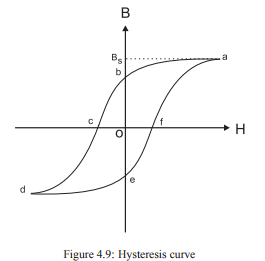When H is varied from its negative maximum value, through zero, to its original positive maximum value then a similar curve defa is traced. Further the complete variation of H from +H to -H and back from -H to +H will trace a closed hysteresis curve abcdef. The area enclosed by the curve abcdef gives the energy loss per cycle per unit volume of the material. This is called as Hysteresis Loss.

Offer running on EduRev: Apply code STAYHOME200 to get INR 200 off on our premium plan EduRev Infinity!

## Engineering Physics - Notes, Videos, MCQs & PPTs

41 videos|48 docs|9 tests

,

,

,

,

,

,

,

,

,

,

,

,

,

,

,

,

,

,

,

,

,

;